# Cross-platform way to enter Unicode characters

The previous post describes the hoops I jumped through to enter Unicode characters on a Mac. Here’s a script to run from the command line that will copy Unicode characters to the system clipboard. It runs anywhere the Python module pyperclip runs.

    #!/usr/bin/env python3

import sys
import pyperclip

cp = sys.argv
ch = eval(f"chr(0x{cp})")
print(ch)
pyperclip.copy(ch)


I called this script U so I could type

    U 03c0

at the command line, for example, it would print π to the command line and also copy it to the clipboard.

Unlike the MacOS solution in the previous post, this works for any Unicode value, i.e. for code points above FFFF.

On my Linux box I had to install xclip before pyperclip would work.

# Curvature at Cairo

I was flipping through Gravitation  this weekend and was curious about an illustration on page 309. This post reproduces that graph.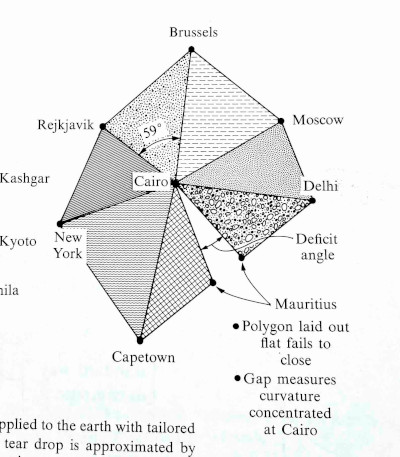The graph is centered at Cairo, Egypt and includes triangles whose side lengths are the distances between cities. The triangles are calculated using only distances, not by measuring angles per se.

The geometry of each triangle is Euclidean: giving the three edge lengths fixes all the features of the figure, including the indicated angle. … The triangles that belong to a given vertex [i.e. Cairo], laid out on a flat surface, fail to meet.

I will reproduce the plot in Python because I’m more familiar with making plots there. But I’ll get the geographic data out of Mathematica, because I know how to do that there.

## Geographic information from Mathematica

I found the distances between the various cities using the GeoDistance function in Mathematica. The arguments to GeoDistance are “entities” which are a bit opaque. When using Mathematica interactively, you can use ctrl + = to enter the name of an entity. There’s some guesswork, e.g. whether I meant New York City or the state of New York when I entered “New York”, but Mathematica guessed correctly. The following code lists the city entities explicitly.

    cities = {
Entity["City", {"Cairo", "Cairo", "Egypt"}],
Entity["City", {"Delhi", "Delhi", "India"}],
Entity["City", {"Moscow", "Moscow", "Russia"}],
Entity["City", {"Brussels", "Brussels", "Belgium"}],
Entity["City", {"Reykjavik", "Hofudhborgarsvaedhi", "Iceland"}],
Entity["City", {"NewYork", "NewYork", "UnitedStates"}],
Entity["City", {"CapeTown", "WesternCape", "SouthAfrica"}],
Entity["City", {"PortLouis", "PortLouis", "Mauritius"}] }


Most of these are predictable, but I would not have guessed the code for Reykjavik or Cape Town. I found these by using the command InputForm and entering the entities as above.

I found the distance from Cairo to each of the other cities with

    Table[GeoDistance[cities[], cities[[i]]], {i, 2, 8}]

and the distances from the cities to their neighbors with

    Table[GeoDistance[cities[[i]], cities[[i + 1]]], {i, 2, 7}]
GeoDistance[cities[], cities[]]


## Drawing the plot

Now that we’ve got the data, how do we draw the plot?

Let’s put Cairo at the origin. First we draw a line from Cairo to Delhi. We might as well put Delhi on the x-axis to make things simple.

Next we need to plot Moscow. We know the distance R1 from Cairo to Moscow, and the distance R2 from Delhi to Moscow. So imagine drawing a circle of radius R1 centered at Cairo and a circle of radius R1 centered at Delhi. Moscow is located where the two circles intersect. The previous post shows how to find the intersection of circles.

The two circles intersect in at two points, so which do we choose? We choose the intersection point that preserves the orientation of the original graph (and the globe). As we go through the cities in counterclockwise order, the cross product of the previous line to the next line should have positive z component.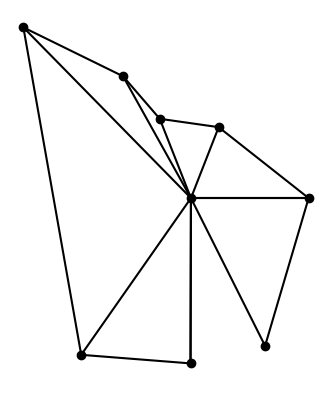This shows that the original graph was not to scale, though the gap between triangles was approximately to scale. In hindsight this should have been obvious: Brussels and Reykjavik are much closer to each other than Capetown and New York are.

## The gap

Why the gap? Because the earth is curved at Cairo (and everywhere else). If the earth were flat, the triangles would fit together without any gaps.

There’s no gap when you take spherical triangles on the globe. But even though the triangles preserve length when projected to the plane, they cannot preserve angles too. The sum of the angles in a spherical triangle adds up to more than 180°, and the amount by which the sum exceeds 180° is proportional to the size of the spherical triangle. Since the angles of triangles in the plane do add up to 180°, each flat triangle fails to capture a bit of the corresponding spherical triangles, and the failures add up to the gap we see in the image.

 Gravitation by Misner, Thorne, and Wheeler. 1973.

# Calculating the intersection of two circles

Given the equations for two circles, how can you tell whether they intersect? And if they do intersect, how do you find the point(s) of intersection?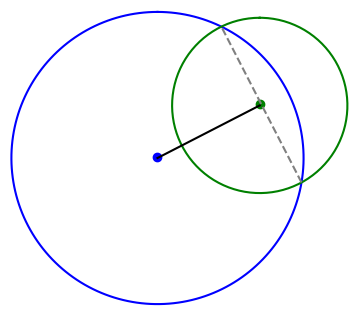MathWorld gives a derivation, but I’d like to add the derivation there in two ways. First, I’d like to be more explicit about the number of solutions. Second, I’d like to make the solution more general.

The derivation begins with the simplifying assumption that one circle is centered at the origin and the other circle is centered somewhere along the x-axis. You can always change coordinates so that this is the case, and doing so simplifies the presentation. Undoing this simplification is implicitly left as an exercise to the reader. I will go through this exercise here because I want a solution I can use in software.

## Finding the x coordinate

Suppose the first circle, the one centered at the origin, has radius R. The other circle is centered at (d, 0) for some d, and has radius r. The  x-coordinate of the intersection is shown to satisfy the following equation.When I got to this point in the derivation I was wondering what assumption was made that guaranteed there is a solution. Clearly if you increase d enough, moving the second circle to the right, the circles won’t intersect. And yet the derivation for x always succeeds, unless d = 0. If d does equal 0, the two circles are concentric. In that case they’re the same circle if R = r; otherwise they never intersect.

## Finding the y coordinate

Why does the derivation for x always succeed even though the intersection might be empty? The resolution depends on the solution for y. What we’ve found is that if the circles intersect, the x coordinate of the point(s) of intersection is given by the equation above.

The y coordinate of the point(s) of intersection satisfiesIf the numerator is negative, there is no real solution, no intersection. If the numerator is zero, there is one solution, and the two circles are tangent. if the numerator is positive, there are two solutions.

The distance between the two intersection points, if there are two intersection points, is a = 2|y|. This will be needed below.## General position

Now suppose we’re first circle is centered at (x0, y0) and the second circle is centered at (x1, y1). Again we let d be the distance between the centers of the circles. The circles intersect twice if d < R + r, once if d = R + r, and never if d > R + r.

Imagine for a moment shifting and rotating the plane so that (x0, y0) goes to the origin and goes to (d, 0). The length of the line segment between the two intersection points is still given by a above. And the distance from the center of the first circle to that line segment is given by the equation for x above.

So to find the points of intersection, we first form a unit vector in the direction of the center of the first circle headed toward the center of the second circle. This is the black line at the top of the post. We move a distance x along this line, with x as in the equation above, and then move perpendicularly a distance a/2 in either direction. This is the dashed gray line.

## Python code

The following code implements the algorithm described above.

    def circle_intersect(x0, y0, r0, x1, y1, r1):
c0 = np.array([x0, y0])
c1 = np.array([x1, y1])
v = c1 - c0
d = np.linalg.norm(v)

if d > r0 + r1 or d == 0:
return None

u = v/np.linalg.norm(v)
xvec = c0 + (d**2 - r1**2 + r0**2)*u/(2*d)

uperp = np.array([u, -u])
a = ((-d+r1-r0)*(-d-r1+r0)*(-d+r1+r0)*(d+r1+r0))**0.5/d
return (xvec + a*uperp/2, xvec - a*uperp/2)


## Application

This post started out to be part of the next post, but it turned out to be big enough to make its own post. The next post looks carefully at an example that illustrates how you could discover that you’re living on a curved surface just by measuring the distances to points around you. I needed the code in this post to make the image in the next post.

# Filtering on how words are being used

Yesterday I wrote about how you could use the spaCy Python library to find proper nouns in a document. Now suppose you want to refine this and find proper nouns that are the subjects of sentences or proper nouns that are direct objects.

This post was motivated by a project in which I needed to pull out company names from a large amount of text, and it was important to know how the company name was being used.

## Dependency labels

Tokens in spaCy have a dependency label attribute dep (or dep_ for its string representation). Dependency labels tell you how a word is being used. For example, dobj tells you the word is being used as a direct object, and nsubj tells you its being used as a nominal subject.

In yesterday’s post the line

    if tok.pos_ == "PROPN":
print(tok)


filtered tokens to look for proper nouns. We could modify the script to also tell us how the proper nouns are being used by printing tok.dep_.

There are three proper nouns in the opening paragraph of Moby Dick: Ishmael, November, and Cato.

Call me Ishmael. … whenever it is a damp, drizzly November in my soul … With a philosophical flourish Cato throws himself upon his sword …

If we run

    if tok.pos_ == "PROPN":
print(tok, tok.dep_)


on the first paragraph we get

    Ishmael oprd
November attr
Cato nsubj


but it’s not obvious what the output means. If we wrap tok.dep_ with spacy.explain we get a more verbose explanation.

    Ishmael object predicate
November attribute
Cato nominal subject


## Pulling out subjects

Now suppose we wanted to pull out words that are subjects. We could filter on tok.dep_ == "nsubj" but there are more kinds of subjects than just nominal subjects. There are six kinds of subjects:

1. nsubj: nominal subject
2. nsubjpass: nominal passive subject
3. csubj: clausal subject
4. csubjpass: clausal passive subject
5. agent: agent
6. expl: expletive

Finding the range of possible values for dependency labels takes some digging. I don’t believe it’s in the spaCy documentation per se, but if you’re persistent you’ll find a link this list or the paper it came from.

# AM over GM

Suppose you take the arithmetic mean and the geometric mean of the first n integers. The ratio of these two means converges to e/2 as n grows . In symbols,Now suppose we wanted to visualize the convergence by plotting the expression on the left side for a sequence of ns.

First let’s let n run from 1 to 100.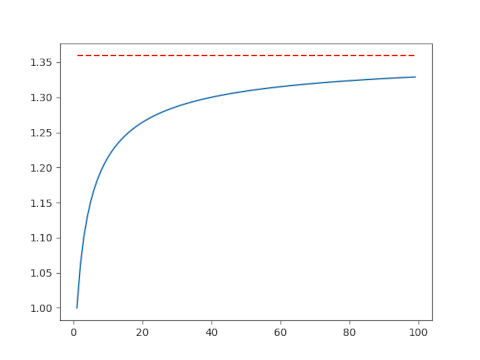This isn’t very convincing. Maybe the convergence is just slow, or maybe it’s not actually converging to e/2. Let’s make another plot including larger values of n. This will require a little additional work.

## Avoiding overflow

Here’s the code that made the plot above.

    import matplotlib.pyplot as plt
import numpy as np
from scipy.special import factorial

AM = lambda n: (n+1)/2
GM = lambda n: factorial(n)**(1/n)
n = np.arange(1, 100)
plt.plot(n, AM(n)/GM(n))
plt.plot(n, 0*n + np.exp(1)/2, 'r--')


This works for n up to 170, but it fails when n = 171 because at that point factorial overflows.

This is a very common situation. We ultimately want to compute a fairly small number, but we encounter extremely large numbers in an intermediate step.

We need to avoid directly calculating n! before taking the nth root. The way to do this is to work with logarithms. Now n! = Γ(n+1) and SciPy has a function for computing the log of the gamma function directly without first computing the gamma function, thus avoiding overflow.

We replace GM above with GM2 below:

    GM2 = lambda n: np.exp(gammaln(n+1)/n)

Now we compute the geometric mean of the first n natural numbers for very large values of n. We only need n = 1000 to see convergence, but the code could handle much larger values of n without overflowing.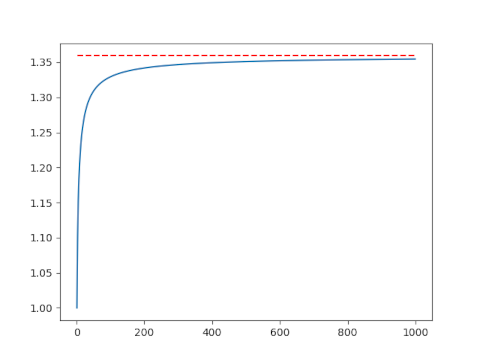## Related posts

 Richard P. Kubelka. Means to an End. Mathematics Magazine, Vol. 74, No. 2 (April 2001), pp. 141–142

# Golden integration

Let φ be the golden ratio. The fractional parts of nφ bounce around in the unit interval in a sort of random way. Technically, the sequence is quasi-random.

Quasi-random sequences are like random sequences but better in the sense that they explore a space more efficiently than random sequences. For this reason, Monte Carlo integration (“integration by darts“) can often be made more efficient by replacing random sequences with quasi-random sequence. This post will illustrate this efficiency advantage in one dimension using the fractional parts of nφ.

Here are functions that will generate our integration points.

    from numpy import random, zeros

def golden_source(n):
phi = (1 + 5**0.5)/2
return (phi*n)%1

def random_source(N):
return random.random()


We will pass both of these generators as arguments to the following function which saves a running average of function evaluates at the generated points.

    def integrator(f, source, N):
runs = zeros(N)
runs = f(source(0))
for n in range(1, N):
runs[n] = runs[n-1] + (f(source(n)) - runs[n-1])/n
return runs


We’ll use as our example integrand f(x) = x² (1 – x)³. The integral of this function over the unit interval is 1/60.

    def f(x):
return x**2 * (1-x)**3
exact = 1/60


Now we call our integrator.

    random.seed(20230429)
N = 1000
golden_run = integrator(f, golden_source, N)
random_run = integrator(f, random_source, N)


Now we plot the difference between each run and the exact value of the integral. Both methods start out with wild fluctuations. We leave out the first 10 elements in order to make the error easier to see.

    import matplotlib.pyplot as plt

k = 10
x = range(N)
plt.plot(x[k:], golden_run[k:] - exact)
plt.plot(x[k:], random_run[k:] - exact)


This produces the following plot.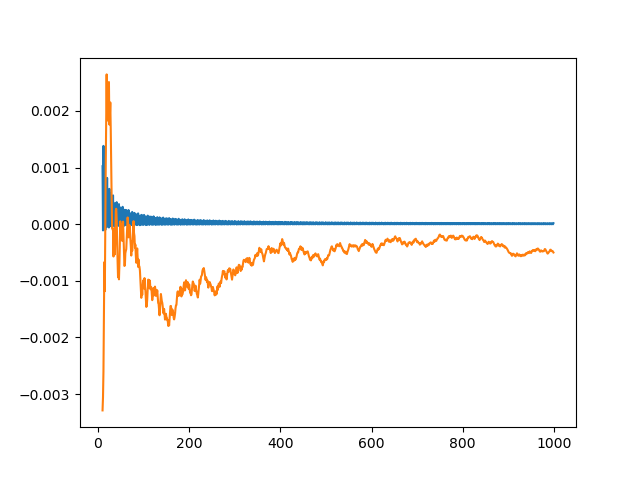The integration error using φn – ⌊φn⌋ is so close to zero that it’s hard to observe its progress. So we plot it again, this time taking the absolute value of the integration error and plotting on a log scale.

    plt.plot(x[k:], abs(golden_run[k:] - exact))
plt.plot(x[k:], abs(random_run[k:] - exact))
plt.yscale("log")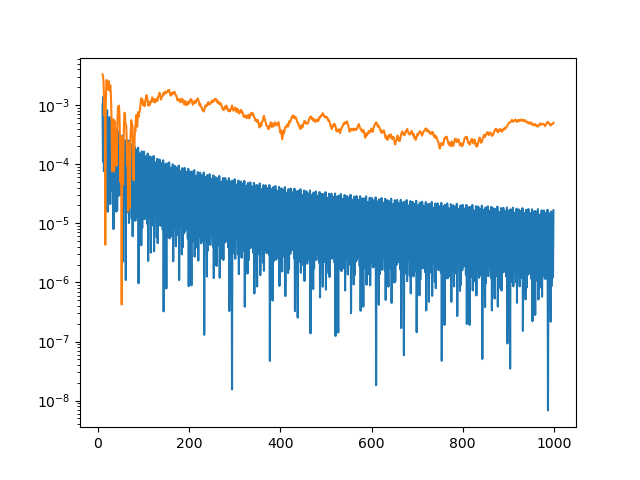The integration error for the golden sequence is at least an order of magnitude smaller, and often a few orders of magnitude smaller.

The function we’ve integrated has a couple features that make integration using quasi-random sequences (QMC, quasi-Monte Carlo) more efficient. First, it’s smooth. If the integrand is jagged, QMC has no advantage over MC. Second, our integrand could be extended smoothly to a periodic function, i.e. f(0) = f(1) and f′(0) = f′(1). This makes QMC integration even more efficient.

# Elliptic functions of a complex argument in Python

I used Mathematica to create the graphics for my previous two posts because SciPy didn’t have the functions I needed. In particular, elliptic integrals and elliptic functions in SciPy only take real-valued arguments, but I needed to use complex arguments. Also, I needed theta functions, which are not in SciPy at all.

I thought mpmath might have the functions I wanted, and indeed it does.

Function names are slightly different between mpmath and SciPy. For example, the ellipe function in mpmath is overloaded to compute the complete elliptic integral of the first kind if given one argument and the incomplete counterpart if given two arguments.

The mpmath library works with arbitrary precision by default, and returns its own numeric types. But you can prefix a function with fp. in order to get a Python floating point value back. For example,

>>> import mpmath as mp
>>> mp.ellipe(1+1j, 1)
mpc(real='1.2984575814159773', imag='0.63496391478473613')
>>> mp.fp.ellipe(1+1j, 1)
(1.2984575814159773+0.634963914784736j)


# When a cubic or quartic has a double root

Thanks to the quadratic equation, it’s easy to tell whether a quadratic equation has a double root. The equationhas a double root if and only if the discriminantis zero.

The discriminant of a cubic is much less known, and the analogs for higher order polynomials are unheard of. There is a discriminant for polynomials of all degrees, though the complexity of the discriminant grows quickly with the degree of the polynomial.

This post will derive the discriminant of a cubic and a quartic.

## Resultants

The resultant of a pair of polynomials is zero if and only if the two polynomials have a common root. Resultants have come up in a couple previous posts about solving trigonometric equations.

A polynomial p(x) has a double root if p and its derivative p‘ are both zero somewhere. The discriminant of p is the resultant of p and p’.

The resultant of two polynomials is a determinant of their Sylvester matrix. This matrix is easier to describe by example than by equation. You basically fill a matrix with shifts of the coefficients of both polynomials and fill in the gaps with zeros.

MathWorld gives the following Mathematica code for the Sylvester matrix of two inputs.

    SylvesterMatrix1[poly1_, poly2_,  var_] :=
Function[{coeffs1, coeffs2}, With[
{l1 = Length[coeffs1], l2 = Length[coeffs2]},
Join[
l1 + l2 -  2], l2 - 2],
l1 + l2 - 2], l1 - 2]
]
]
][
Reverse[CoefficientList[poly1, var]],
Reverse[CoefficientList[poly2, var]]
]


## Cubic discriminant

If we apply this to the cubic polynomialwe get the following matrix.We can compute the resultant by taking the determinant of the above matrix.

    g[x_] := a x^3 + b x^2 + c x + d
SylvesterMatrix1[g[x], D[g[x], x], x]


We get the following resultand we can verify that this is the same result we would get from calling the Resultant directly with

    Resultant[g[x], D[g[x], x], x]

Although the resultant is defined in terms of a determinant, that doesn’t mean that resultants are necessarily computed by computing determinants. The Sylvester matrix is a very special matrix, and there are clever ways to exploit its structure to create more efficient algorithms.

Each term in the resultant has a factor of a, and the discriminant is the resultant divided by –a.

## Quartic discriminant

Now let’s repeat our exercise for the quartic. The Sylvester matrix for the quartic polynomialand its derivative isI created the image above with the following Mathematica code.

    f[x_] := a x^4 + b x^3 + c x^2 + d x + e
TeXForm[SylvesterMatrix1[f[x], D[f[x], x], x]]


If we take the determinant, we get the resultant, but it’s a mess.Again each term has a factor of a, so we can divide by a. to get the discriminant.

If we want to use this in code, we can have Mathematica export the expression in C code using CForm. To generate Python code, it’s more convenient to use FortranForm since Python like Fortran uses ** for exponents.

The following Python code was created by pasting the output of

    FortranForm[Resultant[f[x], D[f[x], x], x]]

and making it into a function.

    def quartic_resultant(a, b, c, d, e):
return a*b**2*c**2*d**2 - 4*a**2*c**3*d**2 - 4*a*b**3*d**3 + 18*a**2*b*c*d**3 \
- 27*a**3*d**4 - 4*a*b**2*c**3*e + 16*a**2*c**4*e + 18*a*b**3*c*d*e \
- 80*a**2*b*c**2*d*e - 6*a**2*b**2*d**2*e + 144*a**3*c*d**2*e \
- 27*a*b**4*e**2 + 144*a**2*b**2*c*e**2 - 128*a**3*c**2*e**2 \
- 192*a**3*b*d*e**2 + 256*a**4*e**3


Let’s try this on a couple examples. Firstwhich has a double root at 0.

As expected

    quartic_resultant(1, -5, 6, 0, 0)

returns 0.

Next let’s tryThe call

    quartic_resultant(1, -10, 35, -50, 24)

returns 144. We expected a non-zero result since our polynomial has distinct roots at 1, 2, 3, and 4.

## Higher order discriminants

In general the discriminant of an nth degree polynomial is the resultant of the polynomial and its derivative, up to a constant. There’s no need to worry about this constant if you’re only concern is whether the discriminant is zero. To get the exact discriminant, you divide the resultant by the leading coefficient of the polynomial and adjust the sign.

The sign convention is a little strange. If you look back at the examples above, we divided by –a in the cubic case but we divided by a in the quartic case. You might reasonably guess that you should divide by a and multiply by (-1)n. But that won’t give you right sign for the quadratic case. The conventional sign is

(-1)n(n – 1)/2.

So when n equals 2 or 3 we get a negative sign, but when n equals 4 we don’t.

# Using Python as a statistical calculator

This post is for someone unfamiliar with SciPy who wants to use Python to do basic statistical calculations. More specifically, this post will look at working with common families of random variables—normal, exponential, gamma, etc.—and how to calculate probabilities with these.

All the statistical functions you need will be in the stats subpackage of SciPy. You could import everything with

    >>> from scipy.stats import *

This will make software developers cringe because it’s good software development practice to only import what you need . But we’re not writing software here; we’re using Python as a calculator.

## Distributions

Every distribution in SciPy has a location parameter loc which defaults to 0, and scale parameter scale that defaults to 1.

The table below lists additional parameters that some common distributions have.

Distribution SciPy name    Parameters
normal norm
exponential expon
beta beta shape1, shape2
binomial binom size, prob
chi-squared chi2 df
F f df1, df2
gamma gamma shape
hypergeometric hypergeom M, n, N
Poisson poisson lambda
Student t t df

When using any statistical software its good to verify that the software is using the parameterization that you expect. For example, the exponential distribution can be parameterized by rate or by scale. SciPy does everything by scale. One way to test the parameterization is to calculate the mean. For example, if the mean of an exp(100) random variable is 100, you’re software is using the scale paraemterization. If the mean is 1/100, it;s using the rate.

## Functions

For a random variable X from one of the families above, we’ll show how to compute Prob(Xx), Prob(Xx), and how to solve for x given one of these probabilities. And for discrete distributions, we’ll show how to find Prob(X = p).

## CDF: Cumulative distribution function

The probability Prob(Xx) is the CDF of X at x. For example, the probability that a standard normal random variable is less than 2 is

    norm.cdf(2)

We didn’t have to specify the location or scale because the standard normal uses default parameters. If X had mean 3 and standard deviation 10 we’d call

    norm.cdf(2, loc=3, scale=10)

For another example, suppose we’re working with a gamma distribution with shape 3 and scale 5 and want to know the probability of such a random variable taking on a value less than 2. Then we’d call

    gamma.cdf(2, 3, scale=5)

The second argument, 2, is the shape parameter.

## CCDF: Complementary cumulative distribution function

The probability Prob(Xx) is the complementary CDF of X at x, which is 1 minus the CDF at x. SciPy uses the name sf for the CCDF. Here “sf” stands for survival function.

Why have both a CDF and CCDF function? One reason is convenience, but a more important reason is numerical accuracy. If a probability p is very near 1, then 1 – p will be partially or completely inaccurate.

For example, let X be a standard normal random variable. The probability that X is greater than 9 is

    norm.sf(9)

which is on the order of 10-19. But if we compute

    1 - norm.cdf(9)

we get exactly 0. Floating point numbers have 16 figures of precision, and we need 19 to represent the difference between our probability and 1. More on why mathematical libraries have functions that seem unnecessary at first here.

## Inverse CDF

SciPy calls the inverse CDF function ppf for percentile point function.

For example, suppose X is a gamma random variable with shape 3 and scale 5, and we want to solve for the value of x such that Prob(Xx) is 0.7. We could do this with

    gamma.ppf(0.7, 3, scale=5)

## Inverse CCDF

SciPy calls the inverse CCDF function isf for inverse survival function. So, for example,

    gamma.isf(0.3, 3, scale=5)

should return the same value as the example above because the point where the right tail has probability 0.3 is the same as the point where the left tail has probability 0.7.

## Probability mass function

For a discrete random variable X we can compute the probability mass function, the probability that X takes on a value x. Unsurprisingly SciPy calls this function pmf.

For example, the probability of seeing 6 heads out of 10 flips of a fair coin is computed by

    binom.pmf(6, 10, 0.5)

## Getting help

To find out more about a probability distribution, call help with that distribution as an argument. For example, you could call

    help(hypergeom)

***

 The reason to not import more than you need is to reduce namespace confusion. If you see a function foo, is that the foo function from package A or from package B. The most common way this comes up in statistics is confusion over the gamma function and the gamma distribution. In SciPy, the gamma function is in scipy.special while the gamma distribution is in scipy.stats. If you’ve imported everything from scipy.stats then gamma means scipy.stats.gamma. You could bring the gamma function into your environment by importing it with another name. For example

    from scipy.special import gamma as gammaf

would import the gamma function giving it the name gammaf.

# Unicode numbers

There are 10 digits in ASCII, and I bet you can guess what they are. In ASCII, a digit is a decimal is a number.

Things are much wilder in Unicode. There are hundreds of decimals, digits, and numeric characters, and they’re different sets.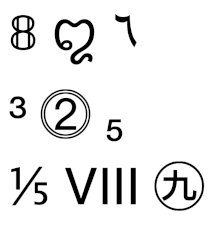The following Python code loops through all possible Unicode characters, extracting the set of decimals, digits, and numbers.

    numbers  = set()
decimals = set()
digits   = set()

for i in range(1, 0x110000):
ch = chr(i)
if ch.isdigit():
if ch.isdecimal():
if ch.isnumeric():


These sets are larger than you may expect. The code

    print(len(decimals), len(digits), len(numbers))

tells us that the size of the three sets are 650, 778, and 1862 respectively.

The following code verifies that decimals are a proper subset of digits and that digits are a proper subset of numerical characters.

    assert(decimals < digits < numbers)

Now let’s look at the characters in the image above. The following code describes what each character is and how it is classified. The first three characters are digits, the next three are decimals but not digits, and the last three are numeric but not decimals.

    from unicodedata import name
for c in "꩓٦":
print(name(c))
assert(c.isdecimal())
for c in "³⓶₅":
print(name(c))
assert(c.isdigit() and not c.isdecimal())
for c in "⅕Ⅷ㊈":
print(name(c))
assert(c.isnumeric() and not c.isdigit())


The names of the characters are

1. MATHEMATICAL DOUBLE-STRUCK DIGIT EIGHT
2. CHAM DIGIT THREE
3. ARABIC-INDIC DIGIT SIX
4. SUPERSCRIPT THREE
5. DOUBLE CIRCLED DIGIT TWO
6. SUBSCRIPT FIVE
7. VULGAR FRACTION ONE FIFTH
8. ROMAN NUMERAL EIGHT
9. CIRCLED IDEOGRAPH NINE

Update: See the next post on ideographic numerals.

Update: There are 142 distinct numbers that correspond to the numerical value associated with a Unicode character. This page gives a list of the values and an example of each value.